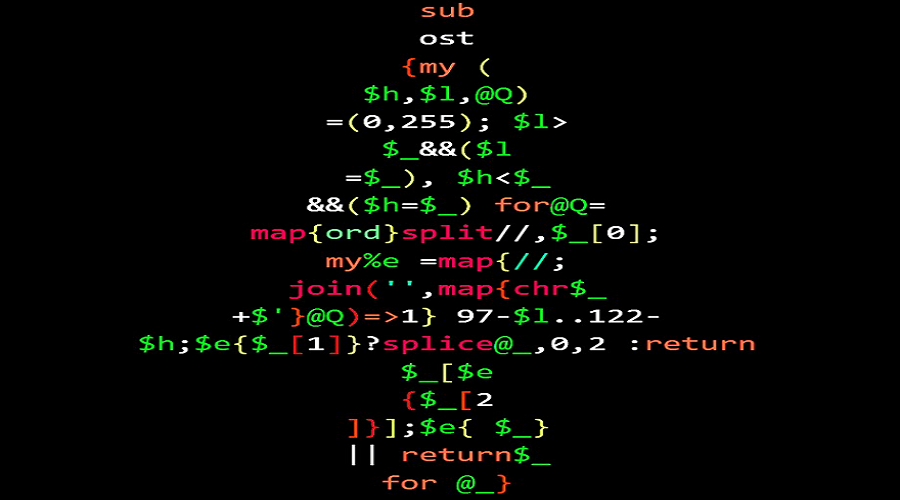## Advent Calendar - December 2, 2022

Friday, Dec 2, 2022| Tags: Perl### |   Day 1   |   Day 2   |   Day 3   |

The gift is presented by `James Smith`. Today he is talking about his solution to “The Weekly Challenge - 193”. This is re-produced for Advent Calendar 2022 from the original post by him.

You are given an integer, `\$n > 0`. Write a script to find all possible binary numbers of size `\$n`.

## Solution

This weeks task 1 is relatively simple. (1) We need to work out what the minimum and maximum integers are and then just print them padded to the right length. Thankfully Perl is great at this as it is integral to it’s design.

The largest value is `'(2^n)-1'` and the template for sprintf is `'%0{n}b'`. Which gives us

``````sub all_binary {                             ## Make a template so we don't have
my \$t = "%0\$_b";                        ## to do interpolation everytime
map { sprintf \$t, \$_ } 0 .. (1<<\$_)-1   ## Need brackets as - is actioned
}
``````

You are given a list of strings of same length, `@s`. Write a script to find the odd string in the given list. Use positional value of alphabet starting with `0, i.e. a = 0, b = 1, ... z = 25`.

## Solution

To find the unique string we note:

If word one isn’t equivalent to word 2 then the word we are looking for is one of these two (the one which doesn’t match the 3rd word) o/w we are looking for the first word that is not equivalent.

### Try 1 - for every string compute a string signature

``````sub sig_str {
my @Q = map { ord \$_ } split //,\$_;
join '', map { chr(96 + \$Q[\$_]-\$Q[\$_+1]) } 0..\$#Q-1
}

sub odd_string_sig {
my \$x = sig_str( \$_ );
return \$_[ \$x eq sig_str( \$_ ) ] if \$x ne sig_str( \$_ );
splice@_,0,2;
\$x eq sig_str( \$_ ) || return \$_ for @_
}
``````

### Try 2 - replace signature with an array ref, here we write an `sig_check` which compares a string against arrayref.

``````sub sig {
my @Q = map { ord \$_ } split //,\$_;
[ map { \$Q[\$_]-\$Q[\$_+1] } 0..\$#Q-1 ]
}

sub sig_check {
my( \$sig, \$str ) = @_;
my @Q = map { ord \$_ } split //,\$str;
\$Q[\$_]-\$Q[\$_+1] == \$sig->[\$_] || return 0 for 0..\$#Q-1;
return 1
}

sub odd_string_sig_check {
my \$x = sig( \$_ );
return \$_[ sig_check( \$x, \$_ ) ] if !sig_check( \$x, \$_ );
splice@_,0,2;
sig_check( \$x, \$_ ) || return \$_ for @_
}
``````

### Try 3… A bit outside in…

We start by working out which are the equivalent words to the first word.

Any word is equivalent if it is in this list… So comparisons are light weight…

``````sub odd_string_eqs {
my @Q = map { ord \$_ } split//,\$_;
my \$l=255;
\$l > \$_ && (\$l=\$_) for @Q;
my \$h=0;
\$h < \$_ && (\$h=\$_) for @Q;
my %eqs = map {
my \$o = \$_;
join( '', map {chr \$_+\$o} @Q ) => 1
} 97-\$l .. 122-\$h;
return \$_[ exists \$eqs{\$_} ]
unless   exists \$eqs{\$_};
splice@_,0,2;
exists \$eqs{\$_} || return \$_ for @_
}
``````

The lines prior to the return - compute this map.

## Performance

How much faster are these… depends on how far along the list you need to go until you find the unique element. Testing a list of strings with the odd one in a random location - we saw:

``````+--------------------+----------+
| Method             | Speed up |
+--------------------+----------+
| signature          |    x 1.0 |
| signature array    |    x 1.4 |
| Equivalent strings |    x 2.5 |
+--------------------+----------+
``````

If you have any suggestion then please do share with us perlweeklychallenge@yahoo.com.

## SO WHAT DO YOU THINK ?

If you have any suggestions or ideas then please do share with us.